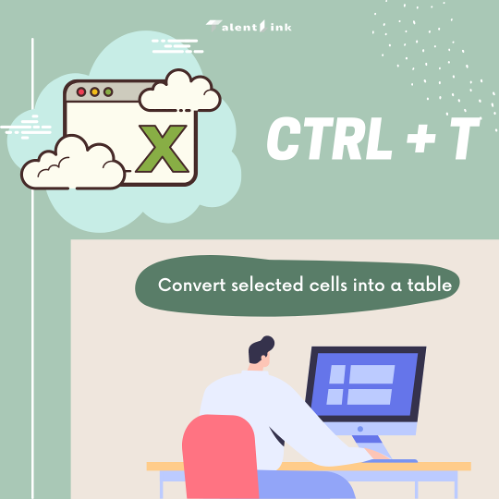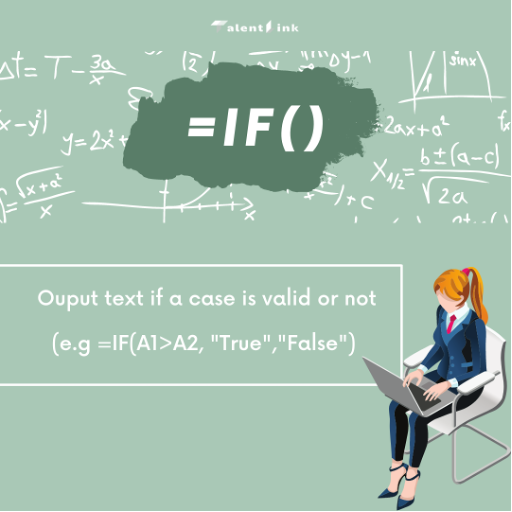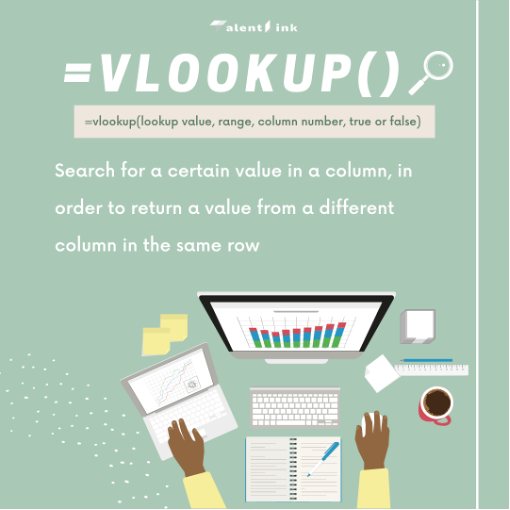top of page
Search

# Excel Cheatsheet

Microsoft Excel remains an indispensable tool, especially for planning and budgeting. Many times, our jobs may even require basic excel knowledge. For people who are not familiar with Excel, utilizing it would seem very tedious and complicated.However, with the shortcuts and formulas, Excel can actually help us save a lot of time and get our work done faster. These are the 6 most useful shortcuts and formulas everyone needs.

1. CTRL + T

Tables are generally required to help us organize and visualize our data clearer. CTRL + T can efficiently help us convert our selected cells into a table.2. F11

Similar to tables, charts can also help us compare and visualize our data better. Bar charts are one of the most used form of charts. By simply clicking F11 we can easily create a bar chart based on the data we have selected.3. =AVERAGE(range:range)

At times where we have a ton of data, it would take up too much time to manually calculate every single one of them. Hence by using the AVG formula, we can easily calculate the average of our selected cells.4. =SUM(range:range)

This is similar to the AVG formula but instead of calculating the average, this formula helps us to add up all the value of our selected cells within seconds.5. =IF()

The IF formula is also one of the most useful Excel formula as it helps us to make logical comparisons between values. Especially when we have a huge set of data, it is almost impossible to manually compare them one by one.6. =vlookup()

This stands for “vertical lookup” and it is a function that makes Excel search for a certain value in a column, in order to return a value from a different column in the same row. Vlookup is especially helpful when we need to classify or categorize data.bottom of page